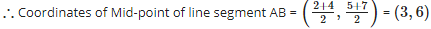Courses

# Test: Coordinate Geometry- 3

## 15 Questions MCQ Test General Aptitude for GATE 2020 | Test: Coordinate Geometry- 3

Description
This mock test of Test: Coordinate Geometry- 3 for Quant helps you for every Quant entrance exam. This contains 15 Multiple Choice Questions for Quant Test: Coordinate Geometry- 3 (mcq) to study with solutions a complete question bank. The solved questions answers in this Test: Coordinate Geometry- 3 quiz give you a good mix of easy questions and tough questions. Quant students definitely take this Test: Coordinate Geometry- 3 exercise for a better result in the exam. You can find other Test: Coordinate Geometry- 3 extra questions, long questions & short questions for Quant on EduRev as well by searching above.
QUESTION: 1

### The distance of the point ( – 3, 4) from the origin is

Solution: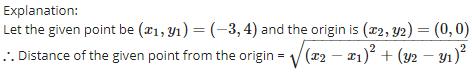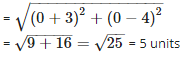QUESTION: 2

### If A and B are the points ( – 6, 7) and ( – 1, – 5) respectively, then the distance 2AB is equal to

Solution: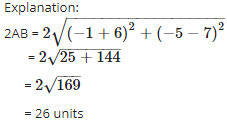QUESTION: 3

### Three consecutive vertices of a parallelogram ABCD are A(1, 2), B(1, 0) and C(4, 0). The co – ordinates of the fourth vertex D are

Solution:

Explanation:

Coordinates are given for  A(1 , 2) , B(1 , 0) and C(4 , 0)

Let coordinates of D be (x,y).
Since diagonals of a parallelogram bisect each other. at point O

Therefore O is the mid point of diagonal AC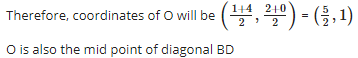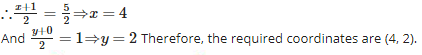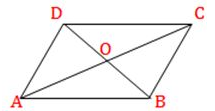QUESTION: 4

The distance between the points ( – 1, – 5) and ( – 6, 7) is

Solution: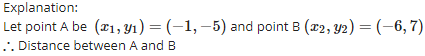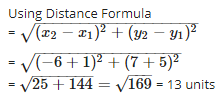QUESTION: 5

The length of the median through A of  ΔABC with vertices A(7, – 3), B(5, 3) and C(3, – 1) is

Solution:

Explanation:

ABC is a triangle with A(7 ,- 3), B(5 , 3) and C(3 , - 1)

Let median on BC bisects BC at D.          (AD is given as the median)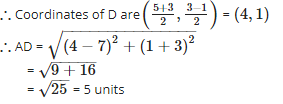QUESTION: 6

If (a, 0) , (0, b) and (x, y) are collinear, then

Solution:

Explanation:

If given points are collinear, the area of triangle formed by these three points is 0.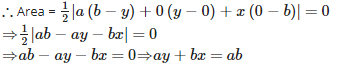QUESTION: 7

If the distance between the points (p, – 5) and (2, 7) is 13 units, then the value of ‘p’ is

Solution:

Explanation:

Let point A be (p,−5) and point B (2, 7) and distance between A and B = 13 units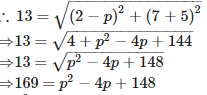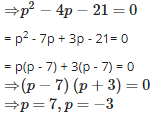QUESTION: 8

The vertices of a quadrilateral are (1, 7), (4, 2), ( – 1, – 1) and ( – 4, 4). The quadrilateral is a

Solution:

Explanation:

Let A (1, 7), B (4, 2), C(−1,−1) and D(−4,4) are the vertices of a quadrilateral ABCD.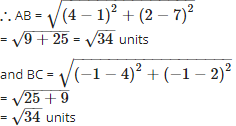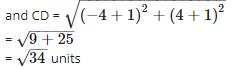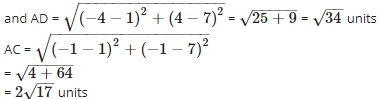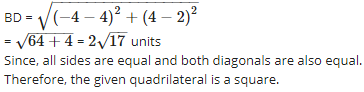QUESTION: 9

The point ( – 3, 5) lies in the ___________ quadrant

Solution:

Explanation:

Since x−coordinate is negative and y−coordinate is positive.Therefore, the point (−3,5) lies in II quadrant.

QUESTION: 10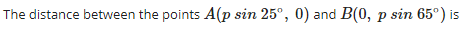Solution:

The sum is according to distance formula
= √(x₂-x₁)²+(y₂-y₁)²
= √(0-p cos 25)² + (p cos 65-0)
= √(p cos 25)² + (p cos  65)²
= √(p cos 25)² + (p sin 25)²
= √(p² cos²25) + (a² sin²25)
= √p²(cos²25 + sin²25)                  we know that  sin²Θ + cos²Θ = 1
= √p²(1)
= √p²
= p Units

QUESTION: 11

The base of an equilateral triangle ABC lies on the y – axis. The co – ordinates of the point C is (0, – 3). If origin is the midpoint of BC, then the co – ordinates of B are

Solution:

Explanation:

Let the coordinate of B be (0,a).
It is given that (0, 0) is the mid-point of BC.

Therefore 0 = (0 + 0) /2 , 0 =(a - 3) /2   a - 3 = 0 ,  a = 3

Therefore, the coordinates of B are (0, 3).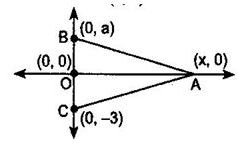QUESTION: 12

If the co – ordinates of a point are (3, – 7), then its ordinate is

Solution:

Explanation:

Since y−coordinate of a point is called ordinate.its distance from the x-axis measured parralel to the  y - axisTherefore, ordinate is −7.

QUESTION: 13

The values of ‘y’ for which the distance between the points (2, – 3) and (10, y) is 10 units is

Solution: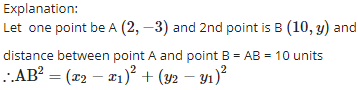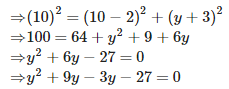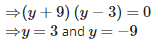QUESTION: 14

If A is point on the x – axis whose abscissa is 5 and B is the point (1, – 3), then the distance AB is

Solution:

Explanation:

Since A is a point on the x - axis, therefore coordinates of A are (5 , 0)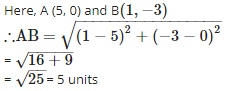QUESTION: 15

The point where the perpendicular bisector of the line segment joining the points A(2, 5) and B(4, 7) cuts is:

Solution:

Explanation:

Since, the point, where the perpendicular bisector of a line segment joining the points A(2 , 5) and B(4 , 7) cuts, is the mid-point of that line segment.